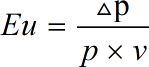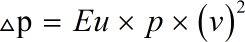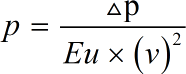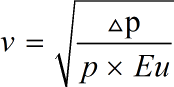Request a Tool

Euler Number Calculator

Euler Number refers to the dimensional number used in calculation of fluid flow

Euler Number
0

Formula• Eu = Euler Number
• Δp = Pressure Change
• p = Density
• v = Characteristic Velocity

Defination / Uses

The link between local pressure drop and kinetic energy per volume is expressed by the Euler Number. The Euler Number is used to describe losses in flow between a local pressure and kinetic energy per volume, with an Euler Number of 1 corresponding to a perfect frictionless flow.

Pressure Change
0

Formula• Δp = Pressure Change
• Eu = Euler Number
• p = Density
• v = Characteristic Velocity

Defination / Uses

The link between local pressure drop and kinetic energy per volume is expressed by the Euler Number. The Euler Number is used to describe losses in flow between a local pressure and kinetic energy per volume, with an Euler Number of 1 corresponding to a perfect frictionless flow.

Density
0

Formula• p = Density
• Eu = Euler Number
• Δp = Pressure Change
• v = Characteristic Velocity

Defination / Uses

The link between local pressure drop and kinetic energy per volume is expressed by the Euler Number. The Euler Number is used to describe losses in flow between a local pressure and kinetic energy per volume, with an Euler Number of 1 corresponding to a perfect frictionless flow.

Output

Characteristic Velocity
0

Formula• v = Characteristic Velocity
• Eu = Euler Number
• Δp = Pressure Change
• p = Density

Defination / Uses

The link between local pressure drop and kinetic energy per volume is expressed by the Euler Number. The Euler Number is used to describe losses in flow between a local pressure and kinetic energy per volume, with an Euler Number of 1 corresponding to a perfect frictionless flow.

How calculte the euler number?

There are only two basic steps.

• First select the option from dropdown. What you need to calculate.
• Then add the other values in their corresponding fields.

Thats it! You will get your answer in less than a second. Use upper given formula in case of manual calculation.

The Euler Number is a dimensional number used in fluid flow calculations. The link between local pressure drop and kinetic energy per volume is expressed by the Euler Number. The Euler Number is used to describe losses in flow between a local pressure and kinetic energy per volume, with an Euler Number of 1 corresponding to a perfect frictionless flow. The Euler Number expresses the link between a local pressure drop produced by a limitation and the kinetic energy per volume of the flow, and is used to characterise energy losses in the flow, with an Euler number of 0 corresponding to a perfect frictionless flow. For quick calculation use our weetools. No sign-up, registration OR captcha is required to use this tool.